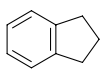# Problem: Consider the compound shown below (a) Give the molecular formula. (b) Give a balanced chemical equation for the combustion.

###### FREE Expert Solution
95% (176 ratings)
###### Problem Details

Consider the compound shown below (a) Give the molecular formula. (b) Give a balanced chemical equation for the combustion.